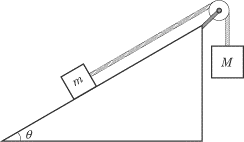• Sho Kano

#### Sho KanoIn a situation like this, there are two equilibrium's,
For just about to accelerate downwards:
mgsinθ - Ffriction - T = 0
For just about to accelerate upwards:
T - Ffriction - mgsinθ = 0

Is there a situation where there is no static friction i.e. when
mgsinθ = T?

Sure. For the equilibrium condition with or without friction, T is always equal to Mg. The m/M ratio is key.In a situation like this, there are two equilibrium's,
For just about to accelerate downwards:
mgsinθ - Ffriction - T = 0
For just about to accelerate upwards:
T - Ffriction - mgsinθ = 0
This are the limiting cases. There are infinitely many equilibriums in between.

Is there a situation where there is no static friction i.e. when
mgsinθ = T?
if m>M
first set angle to 0
then increase angle by tiny amounts and after each increase reset the system to original condition( ie bodies at rest) and check if mass 'm' is at rest
let x = 1st angle at which equilibrium is achieved (body just about to accelerate down)
on further increase , for a small interval of angle , system will stay at rest
let y = 1st angle at which system again starts moving (body just about to accelerate up)
note that on increasing angle above x , tendency of m to go down decreases and to go up increases
on decreasing angle below y , tendency of m to go down increases and to go up decreases
so there must be an angle bet x and y at which both tendency balanced so no static friction needed for equilibrium